1.

2.

3.

4.  $\mathrm{Zero}$

Concept Questions :-

Grouping of cells
High Yielding Test Series + Question Bank - NEET 2020

Difficulty Level:

In the circuit shown in figure find the value of r(internal resistance of the cell) for which power transferred by the cell is maximum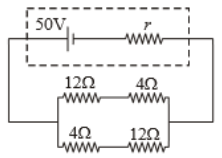1.

2.

3.

4.

Concept Questions :-

Combination of resistors
High Yielding Test Series + Question Bank - NEET 2020

Difficulty Level:

If the voltage across a bulb decreases by 1%, then the percentage change in its power output is :

1.  1%

2.  4%

3.  2%

4.  5%

Concept Questions :-

Heating effect of current
High Yielding Test Series + Question Bank - NEET 2020

Difficulty Level:

1.

2.

3.  $32\mathrm{\Omega }$

4.

Concept Questions :-

Derivation of Ohm's law
High Yielding Test Series + Question Bank - NEET 2020

Difficulty Level:

In the circuit shown in the figure, the current supplied by the battery is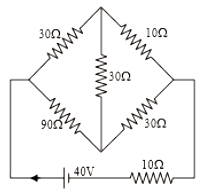1.  2A

2.  1A

3.  0.5A

4.  0.4A

Concept Questions :-

Combination of resistors
High Yielding Test Series + Question Bank - NEET 2020

Difficulty Level:

If a resistance coil is made by joining in parallel two resistances each of 20$\Omega$. An emf of 2V is applied across this coil for 100 seconds. The heat produced in the coil is

(1) 20 J

(2) 10 J

(3) 40 J

(4) 80 J

Concept Questions :-

Heating effect of current
High Yielding Test Series + Question Bank - NEET 2020

Difficulty Level:

1.

2.

3.

4.

Concept Questions :-

Grouping of cells
High Yielding Test Series + Question Bank - NEET 2020

Difficulty Level:

1.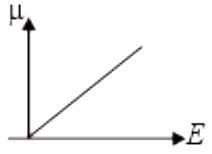2.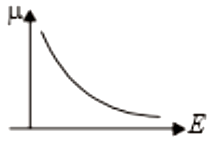3.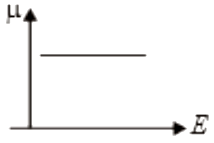4.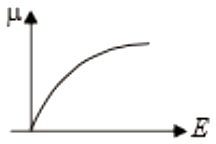Concept Questions :-

Current and current density
High Yielding Test Series + Question Bank - NEET 2020

Difficulty Level:

The equivalent resistance across AB is :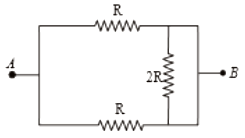1.  2R

2.  R/2

3.  4R/3

4.  3R/2

Concept Questions :-

Combination of resistors
High Yielding Test Series + Question Bank - NEET 2020

Difficulty Level:

An electric Kettle draws 4.2A at 250V. How much time it would take to boil 1kg of water at $20°$C completely to  ?

1.  320 seconds

2.  64 seconds

3.  3840 seconds

4.  960 seconds

Concept Questions :-

Heating effect of current